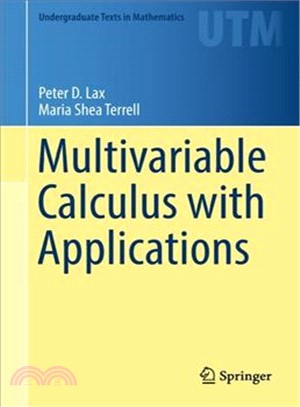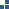瀏覽紀錄

TOP
1/1庫存：1杜威分類Natural sciences & mathematics > Analysis
定  價：NT\$3600元
優惠價： 93240
可得紅利積點：97 點

庫存：1

商品簡介

作者簡介

This text in multivariable calculus fosters comprehension through meaningful explanations. Written with students in mathematics, the physical sciences, and engineering in mind, it extends concepts from single variable calculus such as derivative, integral, and important theorems to partial derivatives, multiple integrals, Stokes’ and divergence theorems. Students with a background in single variable calculus are guided through a variety of problem solving techniques and practice problems.

Examples from the physical sciences are utilized to highlight the essential relationship between calculus and modern science. The symbiotic relationship between science and mathematics is shown by deriving and discussing several conservation laws, and vector calculus is utilized to describe a number of physical theories via partial differential equations. Students will learn that mathematics is the language that enables scientific ideas to be precisely formulated and that science is a source for the development of mathematics.

Peter D. Lax is currently an Emeritus Professor of Mathematics at the Courant Institute of Mathematical Sciences.

Maria Shea Terrell is currently a retired Senior Lecturer in Mathematics at Cornell University.

## 購物須知

為了保護您的權益，「三民網路書店」提供會員七日商品鑑賞期(收到商品為起始日)。

若要辦理退貨，請在商品鑑賞期內寄回，且商品必須是全新狀態與完整包裝(商品、附件、發票、隨貨贈品等)否則恕不接受退貨。

外文館商品之書封，為出版社提供之樣本。實際出貨商品，以出版社所提供之現有版本為主。部份書籍，因出版社供應狀況特殊，匯率將依實際狀況做調整。

無庫存之商品，在您完成訂單程序之後，將以空運的方式為你下單調貨。為了縮短等待的時間，建議您將外文書與其他商品分開下單，以獲得最快的取貨速度，平均調貨時間為1~2個月。